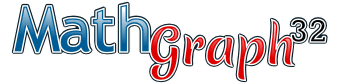Logiciel libre de géométrie, d'analyse et de simulation multiplateforme par Yves Biton

AccueilExemples

# Creation of a 3D figure with MathGraph32 : Example 1

publication lundi 11 avril 2011.

In this example, we will show how to visualize a section of a cube by a plan.

ABCDEFGH is a cube, I is a point of segment [FG], J a point of segment [GH] and K a point of face AEHD. Our goal is to represent the section of plan (IJK) with the faces of the cube in all the possible cases. For this example, you must use version 3.4 of MathGraph32 (version 3.4.1 or later advised). You can download the last version on [this page> 65].

Let us start by creating a virgin figure with the menu File- New figure without unity length. Let us use the menu Constructions - Implement predefined construction. Let us open the directory Space Version2 and choose to implement construction named Frame In Space With Cube. A figure appears with a frame of space. You can on top right of the figure capture the points in fat allowing to turn the frame around the axis (Oz) or to change the slope of this axis. We will use here only the sides of the cube. We thus will use toolto mask point O, the three vectors of color represented starting from O and the three half-lines of origin O.

Let us use the tool Img to create two points linked to segments [FG] and [GH] and the icon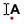to name them I and J.

We now will use the possibility of MathGraph32 to create a point linked to the inside a polygon.

In the color palette, let us activate the blue color. We now use icon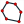to create a polygon joining A, E, H and D (click right when clicked on the fourth point to create the polygon). Now let us click on the tool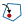. We are asked in the indication line to click initially on the polygon. Once that made, the interior of the polygon flickers and we just have to click inside to create our point. Let us name this point K. We can capture it and move it with the icon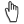but it is bound to the inside of the polygon.

Let us use the toolto mask the polygon. We will now visualize initially part of plan (IJK) corresponding to the inside of this triangle. For that we activate the blue color and the dotted line style. Using the iconlet us create polygon IJK then use the icon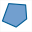to fill the interior of this polygon by a surface. In the color palette, we activate the black color. Using the icon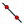let us create lines (IJ) and (EH) and then create their point of intersection Z using the tool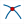.

In the same way let us create line (ZK). If necessary, we will capture K so that line (ZK) is secant with segment [AE], then create the points L and M of intersection of line (LK) with segments [DH] and [AE]. Plan (IJK) must cut the two plans (ABFE) and (CDHG) through two parallel straight lines. We thus create initially line (JL) then with the icon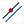the line parallel to (JL) passing through M.

To finish, we create the point N of intersection of this parallel line with segment [BF].

In the color palette, let us activate the blue color (and still keep the dotted line style). Let us use iconto create polygon IJLMN then iconto fill this polygon with a surface.

Here below the figure obtained.

You can capture points I, J and K and make vary $\vartheta$ et $\varphi$